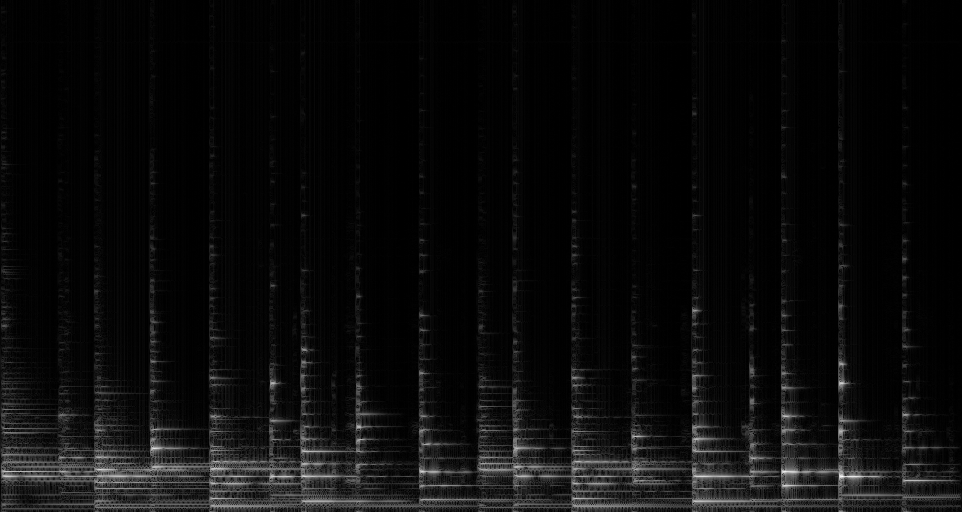# ‘Haskell Spectrograms 2: Array Slicing and Dicing’## So we have a vector

Given a vector of audio, the first step in making a spectrogram is to slice up the audio into frames. This slicing process is defined by the frame size and the hop. The frame size is the number of samples in each frame, the hop is the number of samples between the beginning of adjacent frames. For instance, if slicing the vector [1,2,3,4,5,6,7,8] with a frame size of 4 and a hop size of 2, the resulting frames would be [[1,2,3,4],[3,4,5,6],[5,6,7,8],[7,8,0,0]]. Note that the last frame was padded out with zeros because it extended beyond the original vector.

## Slicing an Array in Haskell

This is where the variety of vector/array libraries in Haskell started to become a bit more of a drag. hsndfile supports Data.StorableVector or Data.Vector as an output type. The haskell-dsp package (available through cabal) uses the standard Haskell Array type. So my first order of business was to convert my freshly-minted StorableVector into an Array.

```import qualified Data.StorableVector as V
import Array as A

arrayFromVector :: V.Vector Double -> A.Array Int Double
arrayFromVector vect =
let l = V.length vect - 1 in
A.array (0, l) (zip [0..l] (V.unpack vect))
```

The above code converts the Vector to a list with V.unpack, then uses the array constructor to create an Array of Double (with Int indexes). Not the most elegant or fast, but effective.

Next we need to take this array and slice it into frames. Lets call that function “getFrames”, which will take a frames size and hop size and give back a list of subarrays of the original array.

```getFrames :: A.Array Int Double -> Int -> Int -> [A.Array Int Double]
getFrames inArr frameSize hop =
[getFrame inArr start frameSize | start  Int -> Int -> A.Array Int Double]
getFrame inVect start length = pad slice length
where
slice = A.ixmap (0, l - 1) (+ start) inVect
l = min length (end - start)
(_,end) = A.bounds inVect
```

Getting a subarray in Haskell is a little bit tricky. The Array library provides an “ixmap” function that takes what you want the bounds of the new array to be, as well as a transformation function to get an index into the old array given an index into the new array. getFrames uses a list comprehension to create a list of slices, each of which is created with getFrame using ixmap. The bounds of the new array are 0 and the length-1, the transformation function to get an index into the original array from the new array is just an offset operation. the pad function comes from the DSP library, available through cabal

## The Furrier[sic] Transform

Once we have ourselves a list of Arrays, the DSP library provides an fft implementation for real signals called rfft, which returns the complex spectrum of an input array. Applying the FFT to each frame in our list is a simple map operation. Here we compose a getFrameMagnitude function with rfft, which takes the complex signal and gives us something that we can plot as a spectrogram.

```getFrameMagnitude :: A.Array Int (Complex Double) -> A.Array Int Double
getFrameMagnitude frame = A.array (0,(l-1)`div`2) \
[(i,log (magnitude (frame!(i+(l-1)`div`2)) + 1)) | i <- [0..((l-1)`div`2)]]
where (_,l) = A.bounds frame
main :: IO ()
main = do
`getFrameMagnitude` looks a little hairy, but the gist is that we’re using list comprehension to create a new list that’s the second half of the input array, where at each sample we take the magnitude, add one to it, then take the log. We add 1 before taking the log so that the log-scaled output will start at 0, for ease of plotting